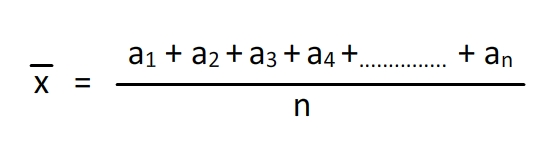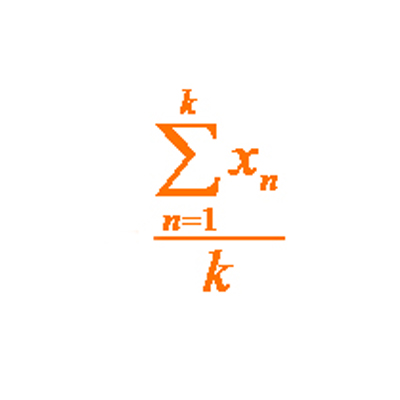﻿ Arithmetic Mean Calculator | Calculate average mean online

# Arithmetic Mean Calculator

#### Formula to Calculate Arithmetic MeanThe arithmetic mean is often known simply as the mean. It is an average, a measure of the centre of a set of data. The arithmetic mean is calculated by adding up all the values and dividing the sum by the total number of values. Arithmetic mean or simply the mean of a variable is defined as the sum of the observations divided by the number of observations.

Mean, also known as the average, is a mathematically calculated number that represents the central tendency of a list of numbers. It is calculated by adding all of the numbers in a list and then dividing by how many numbers are in that list.

The arithmetic mean can be used to compare two sets of data. If one set of data has an arithmetic mean that is greater than the other set’s, it means that the first set has more values higher than any value in the second set.

### Examples of Arithmetic Mean

In this section, we will go through a few examples of arithmetic mean.

The arithmetic mean is the sum of all values in a set of numbers divided by the number of values in the set. The following are some examples of arithmetic mean calculators:

#### For example 1:

If you have the following list: {1, 2, 3} then the arithmetic mean would be (1+2+3) / 3 = 2.

#### Example 2:

The arithmetic mean of 1, 2, 3, 4 and 5 is 2.

## Merits and demerits of Arithmetic mean

#### Merits

• It is rigidly defined.
• It is easy to understand and easy to calculate.
• If the number of items is sufficiently large, it is more accurate and more reliable.
• It is a calculated value and is not based on its position in the series.
• It is possible to calculate even if some of the details of the data are lacking.
• Of all averages, it is affected least by fluctuations of sampling.
• It provides a good basis for comparison.

#### Demerits

• It cannot be obtained by inspection nor located through a frequency graph.
• It cannot be in the study of qualitative phenomena not capable of numerical measurement i.e.Intelligence, beauty, honesty etc.
• It can ignore any single item only at the risk of losing its accuracy.
• It is affected very much by extreme values.
• It cannot be calculated for open-end classes.
• It may lead to fallacious conclusions, if the details of the data from which it is computed are not given.

The arithmetic mean is the simplest calculation to determine the average return. The average return on an asset, observed over a long period of time, is often used as the expected return for future years. Although the future return is unknown and cannot be predicted with great accuracy, the historical average return is as good a guess as any of what the return will be in the future.### Spreading Knowledge Across the World

USA - United States of America  Canada  United Kingdom  Australia  New Zealand  South America  Brazil  Portugal  Netherland  South Africa  Ethiopia  Zambia  Singapore  Malaysia  India  China  UAE - Saudi Arabia  Qatar  Oman  Kuwait  Bahrain  Dubai  Israil  England  Scotland  Norway  Ireland  Denmark  France  Spain  Poland  and  many more....